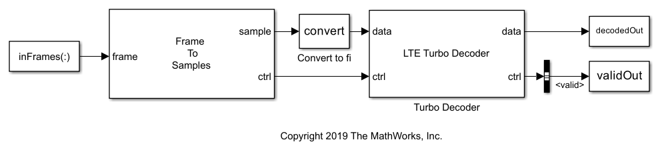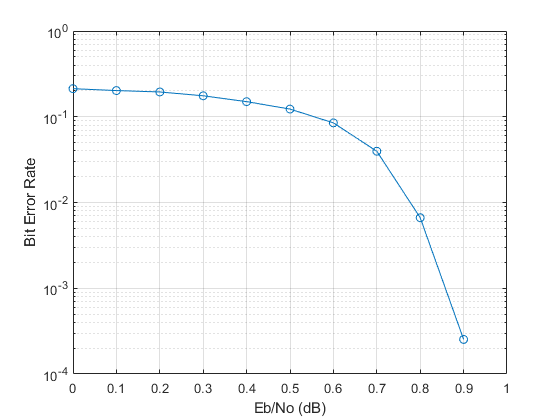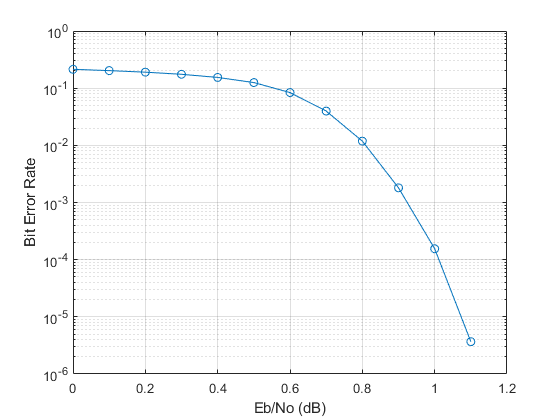# Accelerate BER Measurement for Wireless HDL LTE Turbo Decoder

This example shows the workflow to measure the BER of the Wireless HDL Toolbox™ LTE Turbo Decoder block using parsim to parallelize the simulations across EbNo points. This approach can be used to accelerate other Monte Carlo simulations.

### Introduction

HDL implementations of reference applications are often complex and take a lot of time to simulate. As a result, figuring out the bit error rate (BER) performance by running multiple simulations at different SNR points can be very time consuming. One way to optimize this is to parallelize simulations using the `parsim` command. The `parsim` command runs multiple simulations in parallel when called with a Parallel Computing Toolbox™ license available. This example measures the BER of the LTE Turbo Decoder. To achieve sufficient statistical accuracy, around 100 errors must be obtained at the decoder for each EbNo value. This translates to 1e8 bits at a BER of 10e-6. This type of Monte Carlo simulation is a suitable candidate to parallelize using parsim, where the BER for every EbNo point is performed on workers in parallel.

For every parallel simulation, this example sets up the input data as follows:

1. Generate input data frames

2. Turbo encode

3. QPSK modulate

4. Add AWGN based on the EbNo value

5. Demodulate the noisy symbols

6. Generate soft decisions

The soft decisions become the input to the LTE Turbo Decoder in Simulink®. The turbo decoded bits are compared to the transmitted bits to calculate the BER. Each parallel simulation sends the results back to the main host.

### Configure Parameters and Simulation Objects

The total number of information bits for each `EbNo` point, `bitsPerEbNo`, is divided over multiple simulations, defined by `parsimPerEbNo`. In this way, every simulation runs `bitsPerParsim` bits for a single `EbNo` point. The total number of simulations is `length(EbNo)*parsimPerEbNo`. This example is configured to run only a small number of bits for demonstration purposes. In a real scenario, you must run a sufficient number of samples through the decoder for an accurate measure of the BER at the higher `EbNo` points. When choosing these parameters, consider the memory resources available on the host. A large input data set per simulation or large number of workers could result in slow down or memory exhaustion. The structure `simParam` contains the parameters required for each simulation. This structure is sent to the simulations at a later stage.

```EbNo = 0:0.1:1.1; bitsPerEbNo = 1e5; %1e8; parsimPerEbNo = 2; %10; bitsPerParsim = ceil(bitsPerEbNo/parsimPerEbNo); simParam.blkSize = 6144; simParam.turboIterations = 6; simParam.numFrames = ceil(bitsPerParsim/simParam.blkSize); % frames per simulation simParam.modScheme = 'QPSK'; simParam.bps = 2; % bits per symbol tailBits = 4; % encoder property simParam.encoderRate = simParam.blkSize/(3*(simParam.blkSize+tailBits)); % rate 1/3 Turbo code simParam.samplesizeIn = floor(1/simParam.encoderRate); % 3 samples in at a time simParam.inframeSize = simParam.samplesizeIn*(simParam.blkSize+tailBits); model = 'LTEHDLTurboDecoderBERExample'; open_system(model); ```Start a local parallel pool with minimum of 1 and maximum of `maxNumWorkers`. If a Parallel Computing Toolbox™ license is not available, the simulations will be serialized. The actual size of the pool depends on the number of available cores. Each parallel worker gets assigned one core on which an independent MATLAB® session is launched.

```maxNumWorkers = 3; pool = parpool('local', [1 maxNumWorkers]); ```
```Starting parallel pool (parpool) using the 'local' profile ... Connected to the parallel pool (number of workers: 3). ```

Preallocate a `parsim` object to hold the data required for each simulation. The object can also include handles to functions, which the model calls before or after a simulation. The MATLAB® session on which parsim is executed acts as the main host. The main host is responsible for launching the simulations on the workers, sending the required data to every worker, and receiving the results.

```parsimIn(1:length(EbNo)*parsimPerEbNo) = Simulink.SimulationInput(model); ```

Replicate `EbNo` points to set up `parsimPerEbNo` simulations.

```repEbNo = repmat(EbNo,parsimPerEbNo,1); repEbNo = repEbNo(:); ```

Minimizing data transmission to the workers improves the performance and stability of the main host. Therefore, this example generates the input data in-model, rather than passing the large input data set to each worker. Input data is generated using the pre-simulation function, `presimGenInput` and the BER calculation is also performed in the post-simulation function, `postsimOutput`. These function handles are assigned to each `SimulationInput` object. The post-simulation function is assigned inside the pre-simulation function as shown in the section Pre-Simulation and Post-Simulation Functions.

```for noiseRatio = 1:length(repEbNo) % Calculate the noise variance. EsNo = repEbNo(noiseRatio) + 10*log10(simParam.bps); snrdB = EsNo + 10*log10(simParam.encoderRate); noiseVar = 1./(10.^(snrdB/10)); % Use random but reproducible data. seed = noiseRatio; % For Rapid Accelerator mode, set the simulation % stop time before compilation. parsimIn(noiseRatio) = parsimIn(noiseRatio).setModelParameter('StopTime',num2str(simParam.numFrames)); % Set pre-simulation function. parsimIn(noiseRatio) = parsimIn(noiseRatio).setPreSimFcn(@(simIn) presimGenInput(simIn,noiseVar,seed,simParam)); end ```

Run and show progress of the simulations in the command window. At the end of the simulations, the results are sent back to the main host in an array of structures, `parsimOut`, with one entry created per simulation. Once simulations are complete, shut down the parallel pool.

```parsimOut = parsim(parsimIn,'ShowProgress','on','StopOnError','on'); delete(pool); ```
```[16-Jul-2021 12:34:57] Checking for availability of parallel pool... [16-Jul-2021 12:34:58] Starting Simulink on parallel workers... [16-Jul-2021 12:35:53] Configuring simulation cache folder on parallel workers... [16-Jul-2021 12:35:53] Loading model on parallel workers... [16-Jul-2021 12:36:06] Running simulations... [16-Jul-2021 12:38:49] Completed 1 of 24 simulation runs [16-Jul-2021 12:38:49] Completed 2 of 24 simulation runs [16-Jul-2021 12:38:49] Completed 3 of 24 simulation runs [16-Jul-2021 12:38:56] Completed 4 of 24 simulation runs [16-Jul-2021 12:38:56] Completed 5 of 24 simulation runs [16-Jul-2021 12:38:56] Completed 6 of 24 simulation runs [16-Jul-2021 12:39:03] Completed 7 of 24 simulation runs [16-Jul-2021 12:39:03] Completed 8 of 24 simulation runs [16-Jul-2021 12:39:03] Completed 9 of 24 simulation runs [16-Jul-2021 12:39:09] Completed 10 of 24 simulation runs [16-Jul-2021 12:39:09] Completed 11 of 24 simulation runs [16-Jul-2021 12:39:09] Completed 12 of 24 simulation runs [16-Jul-2021 12:39:15] Completed 13 of 24 simulation runs [16-Jul-2021 12:39:16] Completed 14 of 24 simulation runs [16-Jul-2021 12:39:16] Completed 15 of 24 simulation runs [16-Jul-2021 12:39:21] Completed 16 of 24 simulation runs [16-Jul-2021 12:39:21] Completed 17 of 24 simulation runs [16-Jul-2021 12:39:22] Completed 18 of 24 simulation runs [16-Jul-2021 12:39:27] Completed 19 of 24 simulation runs [16-Jul-2021 12:39:27] Completed 20 of 24 simulation runs [16-Jul-2021 12:39:28] Completed 21 of 24 simulation runs [16-Jul-2021 12:39:33] Completed 22 of 24 simulation runs [16-Jul-2021 12:39:33] Completed 23 of 24 simulation runs [16-Jul-2021 12:39:33] Completed 24 of 24 simulation runs [16-Jul-2021 12:39:33] Cleaning up parallel workers... ```

### Plot BER

Extract the BER values from the array of structures. Combine the BER results for each `EbNo` point and find the average BER per `EbNo` point.

```BER = [parsimOut(:).BER]; BER = transpose(reshape(BER,parsimPerEbNo,length(BER)/parsimPerEbNo)); avgBER = mean(BER,2); semilogy(EbNo,avgBER,'-o'); grid; xlabel('Eb/No (dB)'); ylabel('Bit Error Rate'); ```The plot below shows the results of the BER measurement with `bitsPerEbNo` = 1e8.### Pre-Simulation and Post-Simulation Functions

These functions independently generate input data and process output data for each simulation, which eliminates the need for the main host to store the data in memory for all simulations. The `presimGenInput` function generates input bits, then encodes, modulates and converts them to soft decisions. To make the input frames and parameters available to the model, they are assigned as variables in the global workspace using the `setVariable` function.

```function simIn = presimGenInput(simIn,noiseVar,seed,simParam) rng(seed); % Preallocate arrays for speed. txBits = zeros(simParam.blkSize,simParam.numFrames,'int8'); inFrames = zeros(simParam.inframeSize,simParam.numFrames,'single'); % Generate input frames, turbo encode, modulate and add noise based on % noise variance. for currentFrame = 1:simParam.numFrames txBits(:,currentFrame) = randi([0 1],simParam.blkSize,1); codedData = lteTurboEncode(txBits(:,currentFrame)); txSymbols = lteSymbolModulate(codedData,simParam.modScheme); noise = (sqrt(noiseVar/2))*complex(randn(size(txSymbols)),randn(size(txSymbols))); rxSymbols = txSymbols + noise; inFrames(:,currentFrame) = lteSymbolDemodulate(rxSymbols,simParam.modScheme,'Soft'); end % Set up parameters for Frame to Samples block to serialize data. % Leave sufficient gap between frames. simParam.idleCyclesBetweenSamples = 0; halfIterationLatency = (ceil(simParam.blkSize/32)+3)*32; % window size = 32 algFrameDelay = 2*simParam.turboIterations*halfIterationLatency+(simParam.inframeSize/simParam.samplesizeIn); simParam.idleCyclesBetweenFrames = algFrameDelay; % Assign variables to global workspace. simIn = simIn.setVariable('inFrames',inFrames); simIn = simIn.setVariable('simParam',simParam); % Set post-simulation function and send required data. simIn = simIn.setPostSimFcn(@(simOut) postsimOutput(simOut,txBits,simParam)); end ```

The post-simulation function receives the outputs of the simulation and computes the BER. The results are stored in a structure `results` which parsim returns as `parsimOut`.

```function results = postsimOutput(out, txBits, simParam) decodedOutValid = out.decodedOut(out.validOut); results.numErrors = sum(xor(txBits(:),decodedOutValid)); results.BER = results.numErrors/(simParam.numFrames*simParam.blkSize); end ```

### Conclusion

This example showed how to efficiently measure the BER curve for the Wireless HDL LTE Turbo Decoder block using parsim. If a parallel pool is not used, the linear time to complete the simulations would be approximately 16 hours. As a result of parallelization, the time to run all simulations came down to 5.4 hours, using 3 workers. This was achieved by running the simulations in Rapid Accelerator mode. This workflow can be applied to complex reference applications that require Monte Carlo or other simulations.